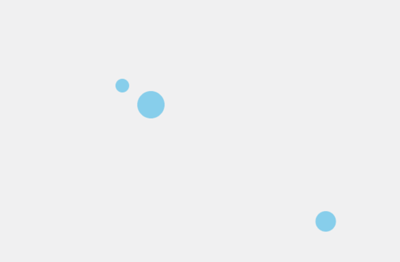canvas基础—简单粒子动画（上）

• canvas
• javascript

## 一、画布概述

canvas是h5新增的组件，它就像一块幕布，可以用js操作它的专属api在上面绘制各种图像和动画，目前兼容到ie9，例如下面这个动画，仅用了四五十行代码就实现了：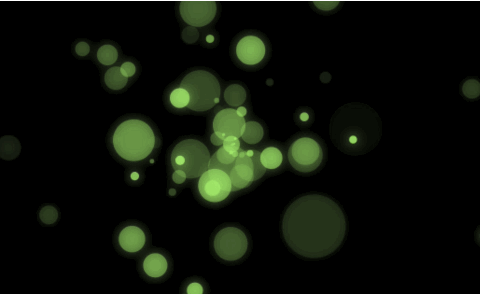## 二、创建画布

canvas在dom结构里是一个标签，有一个默认的宽度。此时注意，如果我们要设置宽度、高度，必须设置在html标签上面或者用js控制，而不能直接设置在css上：
``<canvas id="myCanvas" width="600" height="400" style="background:#f1f1f1;"></canvas><script>    var myCanvas = document.getElementById('myCanvas');    myCanvas.width = 600;    myCanvas.height = 400;</script >``

``// 初始化canvas，获取上下文对象var ctx = myCanvas.getContext('2d');``

get表示得到，context是上下文的意思。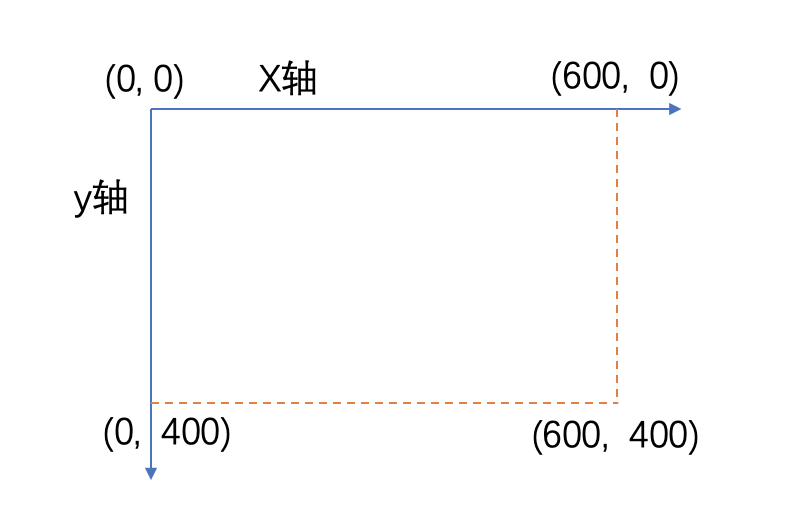## 三、绘制矩形

``// 设置填充的颜色ctx.fillStyle = '#f60';// 绘制实心矩形, 参数意思为在坐标(200,100)的位置，宽高为120*60ctx.fillRect(200, 100, 120, 60);``

``// 设置描边的颜色ctx.strokeStyle = '#f60';// 设置描边粗细, 不设置也默认为1ctx.lineWidth = '1';// 绘制描边矩形, 参数意思为在坐标(200,200)的位置，宽高为120*60ctx.strokeRect(200, 200, 120, 60);``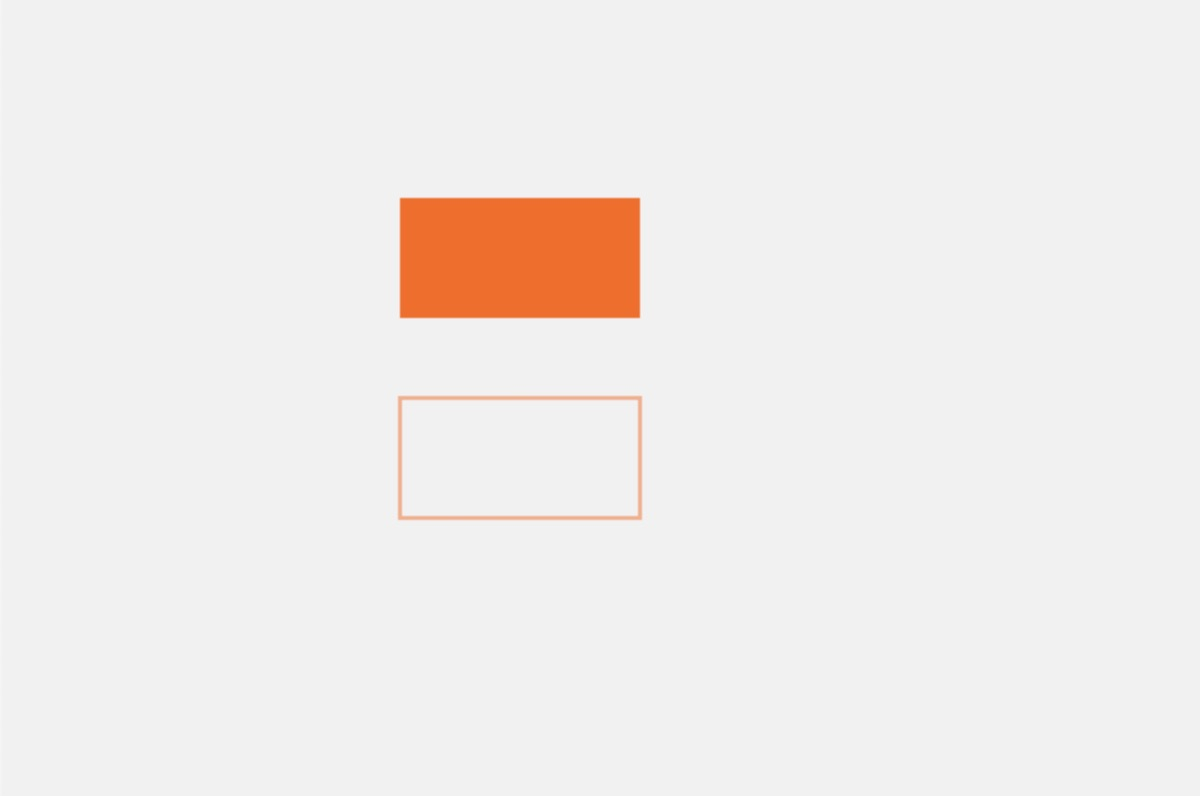## 四、绘制线条和多边形

canvas中可以允许我们绘制自定义的笔触，绘制直线非常简单：

``// 开始画路径ctx.beginPath();// 将画笔移动到一个位置ctx.moveTo(100, 100);// 设置一个点连接上一个位置，画一条线, 暂时看不见ctx.lineTo(150, 150);// 描边渲染出来, 此时可以看见了ctx.stroke();``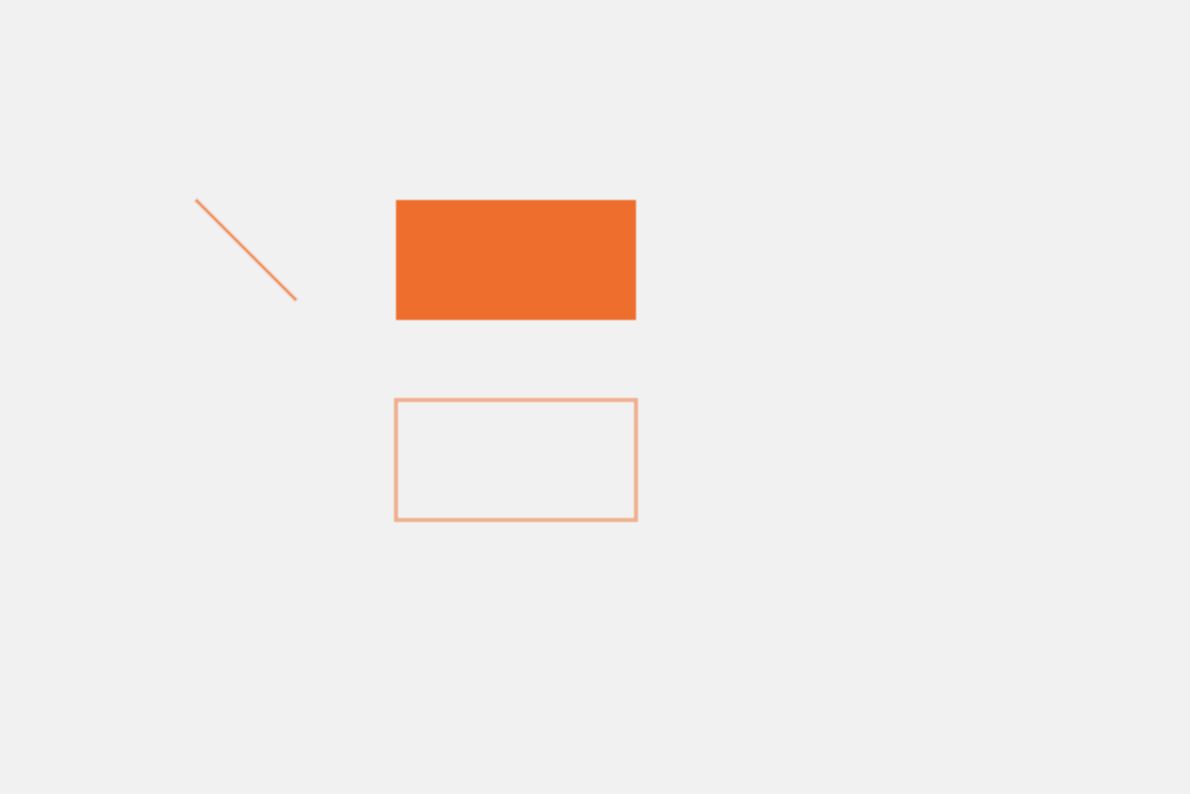``ctx.beginPath();ctx.moveTo(350, 100);ctx.lineTo(500, 200);ctx.lineTo(500, 80);// 最后要返回原点闭合, 或者直接使用ctx.closePath()自动闭合ctx.lineTo(350, 100);// 设置填充的颜色ctx.fillStyle = 'skyblue';// 进行填充ctx.fill();// 设置描边的颜色ctx.strokeStyle = '#000';// 设置描边粗细ctx.lineWidth = '2';// 进行描边ctx.stroke();``

## 五、绘制弧和圆形

``ctx.arc(x, y, radius, startAngle, endAngle, anticlockwise);``

``ctx.beginPath();// 顺时针, -2弧度开始到2弧度结束ctx.arc(100, 330, 40, -2, 2, false);ctx.stroke();    ctx.beginPath();// 逆时针, -2弧度开始到2弧度结束ctx.arc(200, 330, 40, -2, 2, true);ctx.stroke();``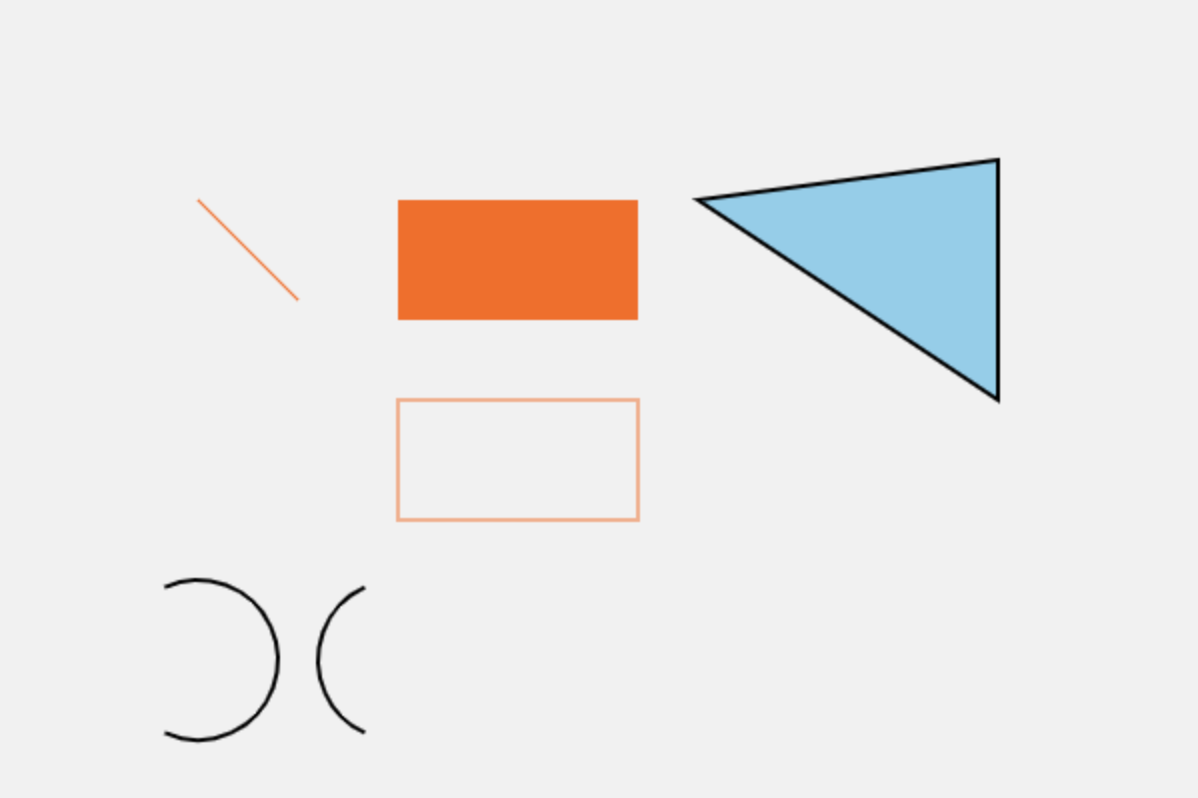``ctx.beginPath();ctx.arc(420, 300, 60, 0, 2 * Math.PI);ctx.fillStyle = 'seagreen';ctx.fill();``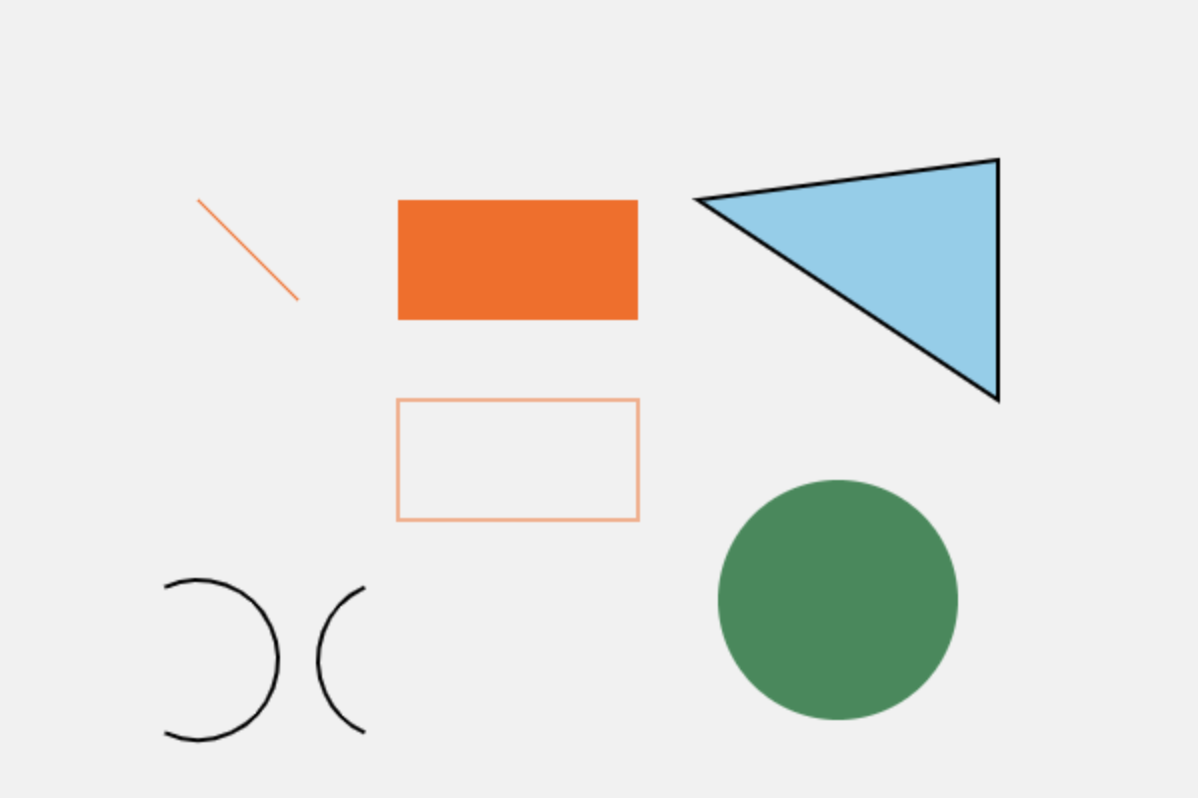## 六、画布的动画和原理

``ctx.clearRect(x, y, w, h);``

``````// 把圆心坐标抽成变量var _x = 30;var _y = 30;// 为画布创建定时器, 一秒50帧setInterval(function() {  // 清屏  ctx.clearRect(0, 0, 600, 400);  ctx.beginPath();  // 画圆  ctx.arc(_x, _y, 15, 0, 2 * Math.PI);  ctx.fillStyle = '#f60';  ctx.fill();  // 画完后改变圆心坐标变量  _x += 6;  _y += 4;  if (_y > 400) {    _x = 30;    _y = 30;  }}, 20)
``````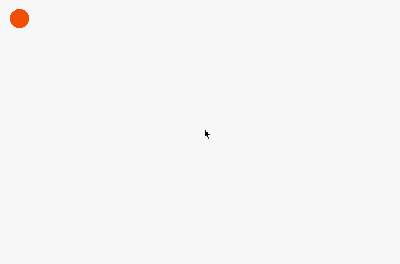## 七、canvas动画与面向对象

``<body>  <canvas id="myCanvas" width="600" height="400" style="background: #f1f1f1;"></canvas>  <script type="text/javascript">    // 获取画布节点    var myCanvas = document.getElementById('myCanvas');    // 设置画布的宽高    myCanvas.width = 600;    myCanvas.height = 400;    // 获取画布的上下文对象    var ctx = myCanvas.getContext('2d');    // 小球的构造函数, 传坐标, 半径和速度形参    function Ball(x, y, r, speed) {      this.x = x;      this.y = y;      this.r = r;      this.speed = speed;      // new出来的时候直接调用下面写的渲染方法把小球放到画布上      this.render();    }    // 渲染小球的方法    Ball.prototype.render = function() {      ctx.beginPath();      ctx.arc(this.x, this.y, this.r, 0, 2 * Math.PI);      ctx.fillStyle = 'skyblue';      ctx.fill();    }    // 更新小球实例的字段的方法    Ball.prototype.update = function() {      this.x += this.speed * 3;      this.y += this.speed * 2;      if (this.y > 400) {        this.x = 30;        this.y = 30;      }    }    // 创建出来的小球实例放在这个数组里    var ballArr = [];    // 每个小球实例的实参都可以不同    ballArr = new Ball(30, 30, 10, 2);    ballArr = new Ball(30, 70, 15, 1.5);    ballArr = new Ball(30, 110, 20, 1);    // 画布唯一定时器, 每秒50帧, 每一帧都先清屏    setInterval(function() {      ctx.clearRect(0, 0, myCanvas.width, myCanvas.height);      for (var i = 0; i < ballArr.length; i++) {        // 每个小球的实例调用自己的update方法来改变自己的坐标字段        ballArr[i].update();        // 每个小球的实例调用自己的render方法来绘制改变坐标后的小球        ballArr[i].render();      }    }, 20);  </script></body>``